BRAIN TEASERS# The Most Difficult Tasks (page 3)

puzzles, riddles, mathematical problems, mastermind, cinemania... These are the tasks listed 21 to 30.

## Decrypt hidden message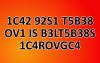Can you decrypt hidden message (1C42 92S1 T5B38 OV1 IS B3LT5B38S 1C4ROVGC4)?
The first user who solved this task is Sanja Šabović.
#brainteasers #wordpuzzles #riddles

## Find number abc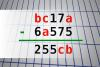If bc17a - 6a575 = 255cb find number abc. Multiple solutions may exist.
The first user who solved this task is Nasrin 24 T.
#brainteasers #math

## MAGIC SQUARE: Calculate A-B*C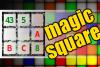The aim is to place the some numbers from the list (5, 8, 10, 13, 16, 18, 37, 40, 43, 45, 67) into the empty squares and squares marked with A, B an C. Sum of each row and column should be equal. All the numbers of the magic square must be different. Find values for A, B, and C. Solution is A-B*C.
The first user who solved this task is Nasrin 24 T.
#brainteasers #math #magicsquare### Todd Barry: Summer School

I never understood the concept of summer school. The teachers going to go up there and go, OK, class, you know that subject you couldnt grasp in nine months? Were going to whip it out in six weeks.
Jokes of the day - Daily updated jokes. New jokes every day.

## Calculate the number 3800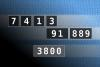NUMBERMANIA: Calculate the number 3800 using numbers [7, 4, 1, 3, 91, 889] and basic arithmetic operations (+, -, *, /). Each of the numbers can be used only once.
The first user who solved this task is Nílton Corrêa de Sousa.
#brainteasers #math #numbermania

## Calculate the number 511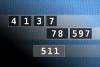NUMBERMANIA: Calculate the number 511 using numbers [4, 1, 3, 7, 78, 597] and basic arithmetic operations (+, -, *, /). Each of the numbers can be used only once.
The first user who solved this task is Nílton Corrêa de Sousa.
#brainteasers #math #numbermania

## Calculate the number 5238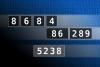NUMBERMANIA: Calculate the number 5238 using numbers [8, 6, 8, 4, 86, 289] and basic arithmetic operations (+, -, *, /). Each of the numbers can be used only once.
The first user who solved this task is Fazil Hashim.
#brainteasers #math #numbermania

## Calculate the number 6890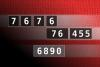NUMBERMANIA: Calculate the number 6890 using numbers [7, 6, 7, 6, 76, 455] and basic arithmetic operations (+, -, *, /). Each of the numbers can be used only once.
The first user who solved this task is Nílton Corrêa de Sousa.
#brainteasers #math #numbermania

## Calculate the number 808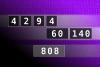NUMBERMANIA: Calculate the number 808 using numbers [4, 2, 9, 4, 60, 140] and basic arithmetic operations (+, -, *, /). Each of the numbers can be used only once.
The first user who solved this task is Nílton Corrêa de Sousa.
#brainteasers #math #numbermania

## Calculate the number 9712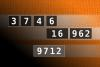NUMBERMANIA: Calculate the number 9712 using numbers [3, 7, 4, 6, 16, 962] and basic arithmetic operations (+, -, *, /). Each of the numbers can be used only once.
The first user who solved this task is Fazil Hashim.
#brainteasers #math #numbermania

## Calculate the number 6705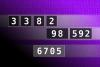NUMBERMANIA: Calculate the number 6705 using numbers [3, 3, 8, 2, 98, 592] and basic arithmetic operations (+, -, *, /). Each of the numbers can be used only once.
The first user who solved this task is Fazil Hashim.
#brainteasers #math #numbermania
 PREVIOUS PAGE NEXT PAGE
 Follow Brain Teasers on social networks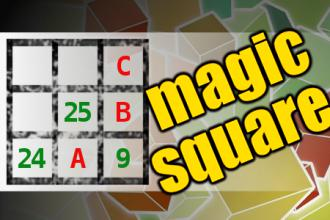The aim is to place the some numbers from the list (8, 9, 14, 24, 25, 30, 45, 46, 51, 89) into the empty squares and squares marked with A, B an C. Sum of each row and column should be equal. All the numbers of the magic square must be different. Find values for A, B, and C. Solution is A+B-C.

## Top 10 Users

 1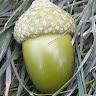H Tav 5048 2Thinh Ddh 5029 3Fazil Hashim 4872 4Alfa Omega 4664 5Djordje Timotijevic 4549 6Mita Kojd 4525 7Jakubovski Vladimir 4443 8Chandu Rajyaguru 4419 9Vladimir Krnac 3952 10Manguexa Wagle 3755
See full ranking list

### Aluminium isolated

In 1886, Charles M. Hall, a young U.S. chemist, completed his electrolytic process for the separation of aluminum from its ore, a mere eight months since he graduated from college. He dissolved the alumina ore in a bath of cryolyte (a mineral containing flourine, sodium and aluminum) and passed electric current through the solution. The process he patented (2 Apr 1889) was the first to become an inexpensive, commercial application. Production began in Nov 1888 by the Pittsburgh Reduction Company started , which later became ALCOA, the Aluminum Company of America.
This site uses cookies to store information on your computer. Some are essential to help the site properly. Others give us insight into how the site is used and help us to optimize the user experience. See our privacy policy.